# Ferroelectric Materials: What Are They? (With Examples)

Contents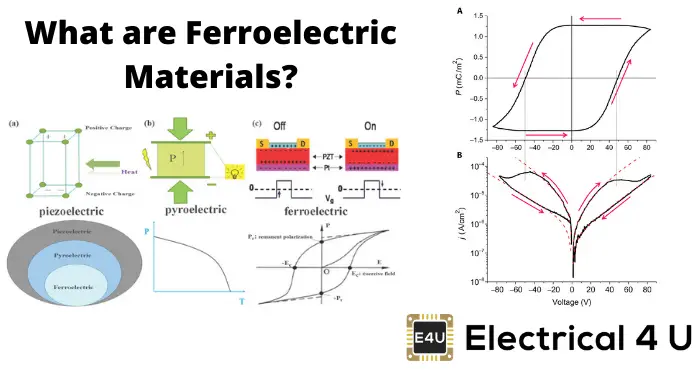## What are Ferroelectric Materials?

Ferroelectric materials are materials that exhibit Ferroelectricity. Ferroelectricity is the ability of the material to have a spontaneous electric polarization. This polarization can be reversed by the application of an external electric field in the opposite direction (figure 1 below). Ferroelectricity (and hence Ferroelectric materials) was discovered by Rochelle salt by Valasek in 1921.

The reversal of polarity of a ferroelectric material via the application of an external electric field is called “switching”.

Ferroelectric materials can maintain the polarisation even once the electric field is removed. Ferroelectric materials have some similarities over ferromagnetic materials, which reveal permanent magnetic moment. The hysteresis loop is almost the same for both materials.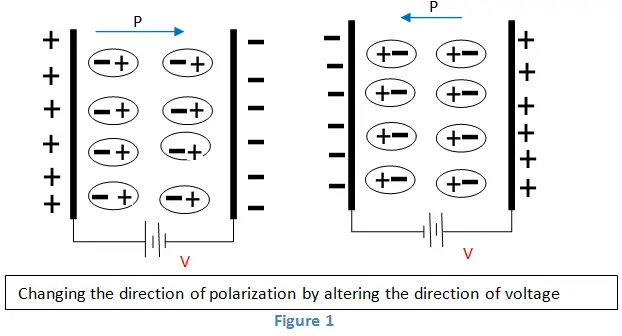Since there are similarities, the prefix is the same for both materials. But all the ferroelectric material must not have Ferro (iron).

All the ferroelectric materials exhibit a piezoelectric effect. The opposed properties of these materials are seen in antiferromagnetic materials.

## Theory of Ferroelectric Materials

The free energy of ferroelectric material based on Ginburg-Landau theory without electric field and any applied stress can be written as Taylor expansion. It is written in terms of P (order parameter) as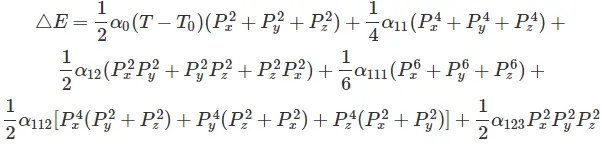(if sixth-order expansion is used)
Px → component of polarization vector, x
Py → component of polarization vector, y
Pz → component of polarization vector, z
αi, αij, αijk → coefficients should be constant with the crystal symmetry.
α0 > 0, α111> 0 → for all ferroelectrics
α11< 0 → ferroelectrics with the first-order transition
α0 > 0 → ferroelectrics with second-order transition

For investigating different phenomena and domain formation in ferroelectrics, these equations are used in the phase-field model.

Usually, it is used by adding some terms such as an elastic term, a gradient term, and an electrostatic term to this free energy equation.

Using the finite difference method, the equations are solved subject to Linear elasticity and Gauss’s law constraints.

A cubic to the tetragonal phase transition of spontaneous polarization of a ferroelectric can be obtained from the expression for free energy.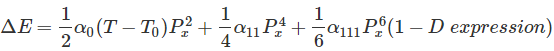It has a character of dual well potential with double energy minima at P = ± Ps.
Ps → spontaneous polarization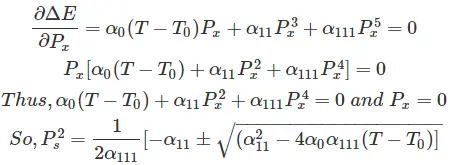By simplifying, eliminating the negative root, and substitute α11 = 0 we get,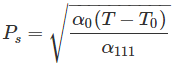## Polarization and Hysteresis Loop

First, we take a dielectric material, and a peripheral electric field is given. We can see that the polarization will always be directly proportional to the applied field, represented in figure 2.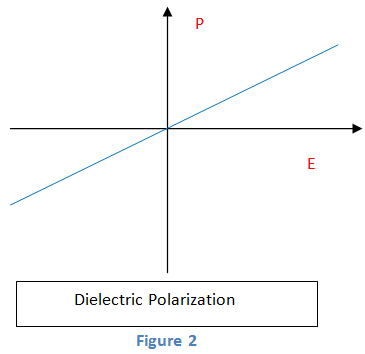Next, when we polarise a paraelectric material, we get a nonlinear polarization. However, it is a function of the field, as shown in figure 3.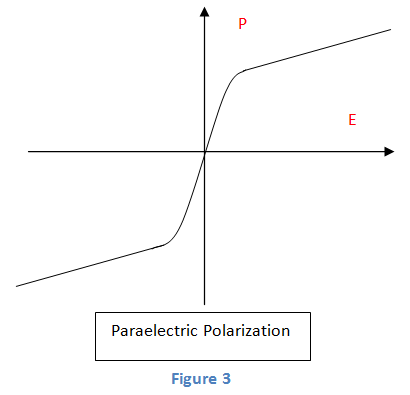Next, we take a ferroelectric material, and an electric field is given to it. We get a nonlinear polarization.

It also exhibits nonzero spontaneous polarization without a peripheral field.

We can also see that by inverting the direction of the applied electrical field, the direction of polarization can be inverted or changed.

Thus, we can say that the polarization will depend on the present and the previous condition of the electric field. The hysteresis loop is obtained as in figure 4.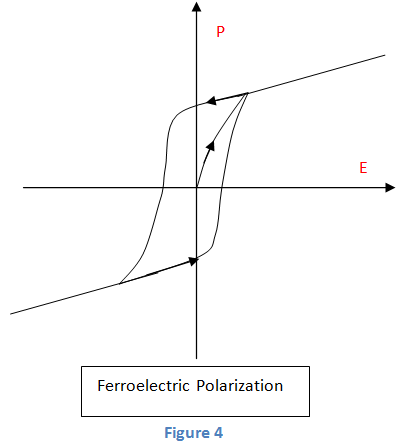## Curie Temperature

The properties of these materials exist only below a definite phase conversion temperature. Above this temperature, the material will become paraelectric materials.

That is, loss in spontaneous polarization. This definite temperature is called Curie temperature (TC).

Most of these materials above Tc will lose the piezoelectric property as well.

The variation of a dielectric constant using temperature in the non-polar, paraelectric state is shown by Curie-Weiss law as given below.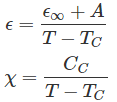ε → Dielectric constant
ε → ε at temperature, T >> TC
A → Constant
TC → Curie point
T → Temperature
χ → Susceptibility
CC → Curie constant of the material

The dielectric constant and temperature characteristic of a ferroelectric material is represented below.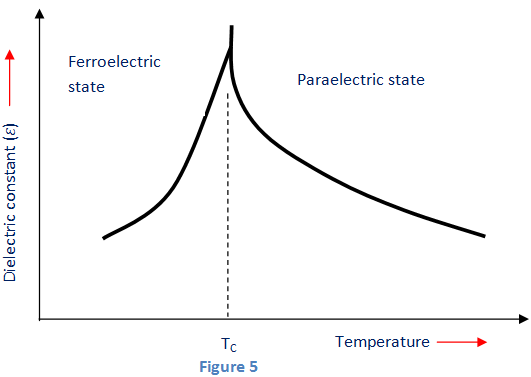## Examples of Ferroelectric Materials

Examples of Ferroelectric materials:

• BaTiO3
• PbTiO3
• Triglycine Sulphate
• PVDF
• Lithium tantalite etc.

## Application of Ferroelectric Materials

Ferroelectric materials have many applications, including:

Want To Learn Faster? 🎓
Get electrical articles delivered to your inbox every week.
No credit card required—it’s 100% free.